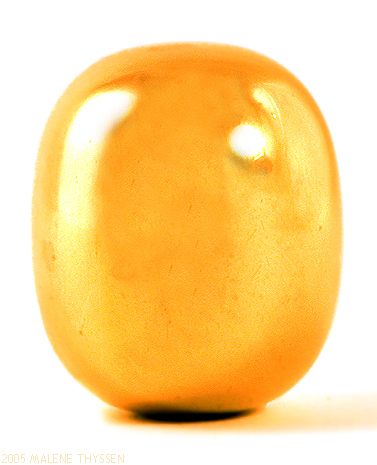# Superegg

How optimising the space around a circular monument is related to supereggsImage: Holger.Ellgaard, CC BY-SA 3.0

Let me take you to centre of Stockholm—back in 1959. The capital of Sweden was being rebuilt after World War II. As part of this program, two big arteries had been built and they intersected in the heart of the city: Sergels torg (Sergel’s Square). This place appeared to be a big problem for city planners, as its shape wasn’t really a square, but a rectangle.

The idea was to plan a roundabout around it. But what shape should this roundabout have? A circle?Such a waste of space! Ellipse?Too narrow for the traffic in the red parts.

Desperate times call for desperate measures, ie for mathematicians. More precisely, the team of architects asked for help from Piet Hein, a Danish mathematician, inventor, designer, author, and poet (what else?!). And he invented a superellipse.

To simplify the task, let’s start with a circle. Just a standard $x^2+y^2=1$—nothing fancy.Have you ever wondered what happens if we enlarge the exponents in this familiar equation? So what does, for example, $x^6+y^6=1$ look like?Is it a circle or a square? Or maybe a squircle? The higher the exponent, the closer to a square we get: $x^{100}+y^{100}=1$ is almost indistinguishable from a square.Now let’s complicate things, just a little bit, and look at a more general shape: an ellipse. For example, $\left(\frac{x}{3}\right)^2+\left(\frac{y}{2}\right)^2=1$ looks like thisAgain, as we enlarge the exponents, we get closer and closer to a rectangle. So here’s $\left(\frac{x}{3}\right)^{10}+\left(\frac{y}{2}\right)^{10}=1$and $\left(\frac{x}{3}\right)^{100}+\left(\frac{y}{2}\right)^{100}=1$Almost a rectangle, right? These interesting shapes are called superellipses.

Remember that our original problem was to build a roundabout rectangular enough to fill most of the available space and circular enough to allow for smooth traffic flow. Hein found out that the perfect shape was an ellipse with exponent equal 2.5 (precisely, the Sergels torg roundabout can be described by $\left(\frac{x}{6}\right)^{2.5}+\left(\frac{y}{5}\right)^{2.5}=1$).

Superellipses quickly became popular among architects all over the world. For example, the famous Mexican Azteca Olympic Stadium has such a shape.

Other applications include:

• A possible shape of the table for negotiators after the Vietnam War, as a compromise between a circular one (“everyone’s equal”) and a rectangular one (“two sides on the conflict”).  Unfortunately, this amazing idea was rejected.
• The Tobler hyperelliptical projection (a method for drawing maps) uses arcs of superellipses as meridians.
• In mobile operating systems, iOS app icons have superellipse curves.

Don’t get impatient, I haven’t forgotten about Easter! Take your favourite superllipse, rotate it along its longest axis and you’ll get a superegg! Why is it so super? Well, it can stand upright on a flat surface or on top of another superegg. This is a proper solution to the egg of Columbus problem!Brass superegg by Piet Hein. Image: Malene, CC BY-SA 3.0

Happy Easter!As a PhD student at Imperial College, London, Paula uses maths and stats to study the impact of wind energy on electricity prices. The title of her TEDx talk `Let’s have a maths party!’ seems to summarise her two favourite activities.
@PaulaRowinska    paularowinska.wordpress.com/    + More articles by Paula